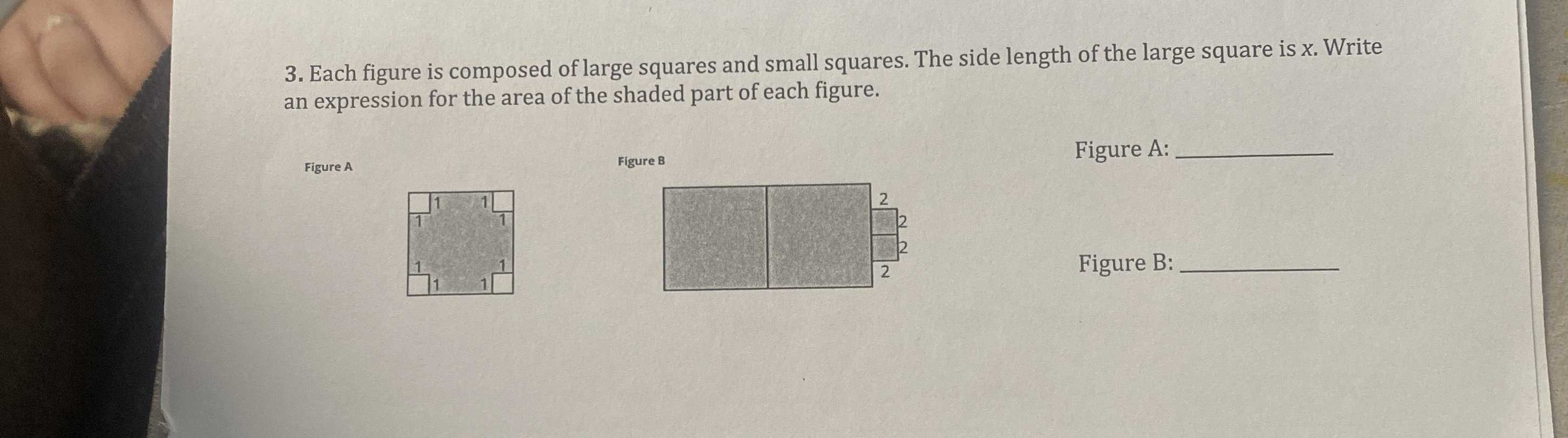### ¿Todavía tienes preguntas de matemáticas?

Pregunte a nuestros tutores expertos
Algebra
Pregunta3. Each figure is composed of large squares and small squares. The side length of the large square is $$x$$ . Write an expression for the area of the shaded part of each figure.

Figure A: Figure B: Figure A: Figure B:

Figure a) $$x^{2}- 4$$
Figure b) $$2x^{2}+ 8$$